# From CAPM To Hedging

This is a provocative question. Patrick was clever to disallow Berkshire. In this post, we are going to use this question to launch into the basics of regression, correlation, beta hedging and risk.

Let’s begin.

## My Reaction To The Question

I don’t know anything about picking stocks. I do know about the nature of stocks which makes this question scary. Why?

1. Stocks don’t last forever

Many stocks go to zero. The distribution of many stocks is positively skewed which means there’s a small chance of them going to the moon and reasonable chance that they go belly-up. The price of a stock reflects its mathematical expectation. Since the downside is bounded by zero and the upside is infinite, for the expectation to balance the probability of the stock going down can be much higher than our flawed memories would guess. Stock indices automatically rebalance, shedding companies that lose relevance and value. So the idea that stocks up over time is really stock indices go up over a time, even though individual stocks have a nasty habit of going to zero. For more see Is There Actually An Equity Premium Puzzle?.

2. Diversification is the only free lunch

The first point hinted at my concern with the question. I want to be diversified. Markets do not pay you for non-systematic risk. In other words, you do not get paid for risks that you can hedge. All but the most fundamental risks can be hedged with diversification. See Why You Don’t Get Paid For Diversifiable Risks. To understand how diversifiable risks get arbed out of the market ask yourself who the most efficient holder of a particular idiosyncratic risk is? If it’s not you, then you are being outbid by someone else, or you’re holding the risk at a price that doesn’t make sense given your portfolio choices. Read You Don’t See The Whole Picture to see why.

My concerns reveal why Berkshire would be an obvious choice. Patrick ruled it out to make the question much harder. Berkshire is a giant conglomerate. Many would have chosen it because it’s run by masterful investors Warren Buffet and Charlie Munger. But I would have chosen it because it’s diversified. It is one of the closest companies I could find to an equity index. Many people look at the question and think about where their return is going to be highest. I have no edge in that game. Instead, I want to minimize my risk by diversifying and accepting the market’s compensation for accepting broad equity exposure.

In a sense, this question reminds me of an interview question I’ve heard.

You are gifted $1,000,000 dollars. You must put it all in play on a roulette wheel. What do you do? The roulette wheel has negative edge no matter what you do. Your betting strategy can only alter the distribution. You can be crazy and bet it all on one number. Your expectancy is negative but the payoff is positively skewed…you probably lose your money but have a tiny chance at becoming super-rich. You can try to play it safe by risking your money on most of the numbers, but that is still negative expectancy. The skew flips to negative. You probably win, but there’s a small chance of losing most of your gifted cash. I would choose what’s known as a minimax strategy which seeks to minimize the maximum loss. I would spread my money evenly on all the numbers, accept a sure loss of 5.26%.1 The minimax response to Patrick’s question is to find the stock that is the most internally diversified. ## Berkshire Vs The Market I don’t have an answer to Patrick’s question. Feel free to explore the speculative responses in the thread. Instead, I want to dive further into my gut reaction that Berkshire would be a reasonable proxy to the market. If we look at the mean of its annual returns from 1965 to 2001, the numbers are gaudy. Its CAGR was 26.6% vs the SP500 at 11%. Different era. Finding opportunities at the scale Buffet needs to move the needle has been much harder in the past 2 decades.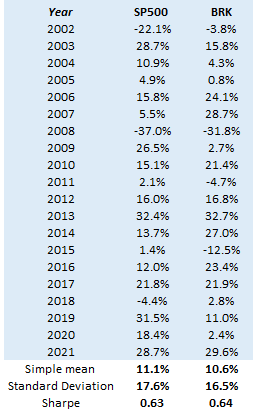Buffet has been human for the past 20 years. This is a safer assumption than the hero stats he was putting up in the last half of the 20th century. The mean arithmetic returns and standard deviations validate my hunch that Berkshire’s size and diversification 2 make it behave like the whole market in a single stock. Let’s add a scatterplot with a regression.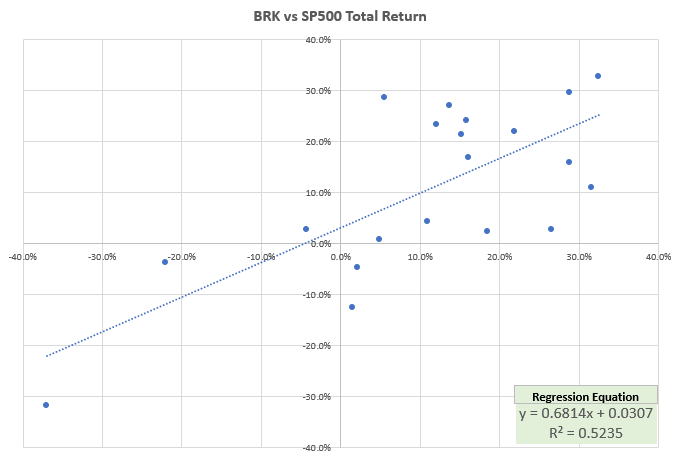If you tried to anticipate Berkshire’s return, your best guess might be its past 20 year return, distributed similarly to its prior volatility. Another approach would be to see this relationship to the SP500 and notice that a portion of its return can simply be explained by the market. It clearly has a positive correlation to the SP500. But just how much of the relationship is explained by SP500? This is a large question with practical applications. Specifically, it underpins how market netural traders think about hedges. If I hedge an exposure to Y with X how much risk do I have remaining? To answer this question we will go on a little learning journey: 1. Deriving sensitivities from regressions in general 2. Interpreting the regression 3. CAPM: Applying regression to compute the “risk remaining of a hedge” On this journey you can expect to learn the difference between beta and correlation, build intuition for how regressions work, and see how market exposures are hedged. ## Unpacking The Berkshire Vs SP500 Regression A regression is simply a model of how an independent variable influences a dependant variable. Use a regression when you believe there is a causal relationship between 2 variables. Spurious correlations are correlations that will appear to be causal because they can be tight. The regression math may even suggest that’s the case. I’m sorry. Math is a just a tool. It requires judgement. The sheer number of measurable quanitites in the world guarantees an infinite list of correlations that serve as humor not insight3. The SP500 is steered by the corporate earnings of the largest public companies (and in the long-run the Main Street economy4) discounted by some risk-aware consensus. Berkshire is big and broad enough to inherit the same drivers. We accept that Berkshire’s returns are partly driven by the market and partly due to its own idiosyncracies. Satisfied that some of Berkshire’s returns are attributable to the broader market, we can use regression to understand the relationship. In the figure above, I had Excel simply draw a line that best fit the scatterplot with SP500 being the independent variable, or X, and Berkshire returns being the dependant or Y. The best fit line (there are many kinds of regression but we are using a simple linear regression) is defined the same way in line is: by a slope and an intercept. The regression equation should remind you of the generic form of a line y = mx + b where m is the slope and b is the intercept. In a regression: y=α+βx where: y = dependant variable (Berkshire returns) x = independent variable (SP500 returns) α = the intercept (a constant) β = the slope or sensitivity of the Y variable based on the X variable If you right-click on a scatterplot in Excel you can choose “Add Trendline”. It will open the below menu where you can set the fitted line to be linear and also check a box to “Display Equation on chart”.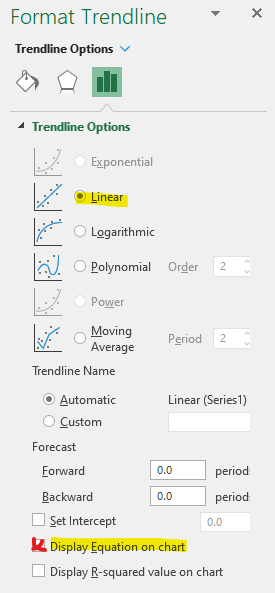This is how I found the slope and intercept for the Berkshire chart: y = .6814x + .0307 Suppose the market returns 2%: Predicted Berkshire return = .6814 * 2% + 3.07% Predicted Berkshire return = 4.43% So based on actual data, we built a simple model of Berkshire’s returns as a function of the market. It’s worth slowing down to understand how this line is being created. Conceptually it is the line that minimizes the squared errors between itself and the actual data. Since each point has 2 coordinates, we are dealing with the variance of a joint distribution. We use covariance instead of variance but the concepts are analogous. With variance we square the deviations from a mean. For covariance, we multiply the distance of each X and Y in a coordinate from their respective means: (xᵢ – x̄)(yᵢ -ȳ) Armed with that idea, we can compute the regression line by hand with the following formulas: β or slope = covar(x,y)/ var(x) α or intercept = ȳ – β̄x̄ We will look at the full table of this computation later to verify Excel’s regression line. Before we do that, let’s make sure that this model is even helpful. One standard we could use to determine if the model is useful is if it performs better than the cheapest naive model that says: Our predicted Berkshire return simply is mean return from sample. This green arrows in this picture represent the error between this simple model and the actual returns.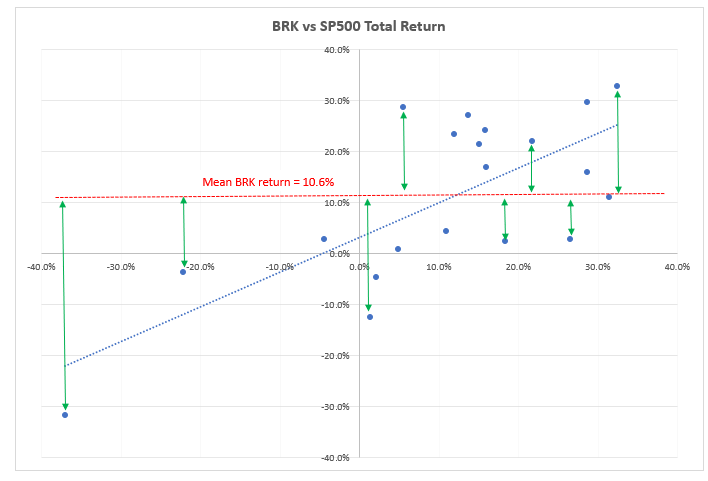This naive model of summing the squared differences from the mean of Berkshire’s returns is exactly the same as variance. You are computing squared differences from a mean. If you take square root of the average of the squared differences you get a standard deviation. In, this simple model where our prediction is simply the mean our volatility is 16.5% or the volatility of Berkshire’s returns for 20 years. In the regression context, the total variance of the dependent variable from its mean is knows as the Total Sum of Squares or TSS The point of using regression though is we can make a better prediction of Berkshire’s returns if we know the SP500’s returns. So we can compare the mean to the fitted line instead of the actual returns. The sum of those squared differences is known as the Regression Sum Of Squares or RSS. This is the sum of squared deviations between the mean and fitted predictions instead of the actual returns. If there is tremendous overlap between the RSS and TSS, than we think much of the variance in X explains the variance of Y. The last quantity we can look at is the Error Sum of Squares or ESS. These are the deviations from the actual data to the predicted values represented by our fitted line. This represents the unexplained portion of Y’s variance.Let’s use 2008’s giant negative return to show how TSS, RSS, and ESS relate.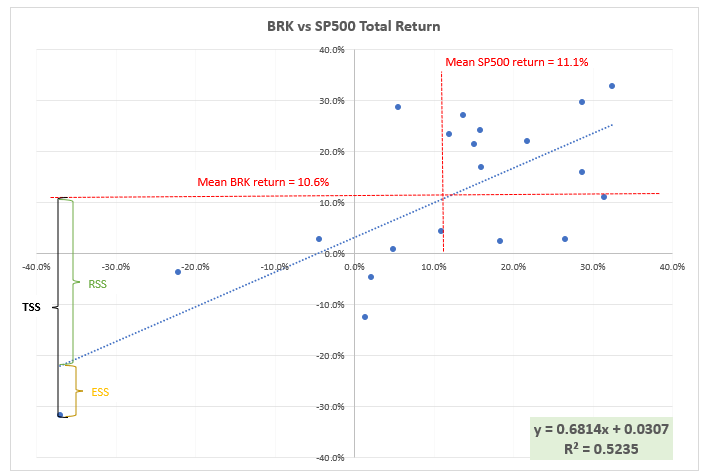The visual shows: TSS = RSS + ESS We can compute the sum of these squared deviations simply from their definitions:  TSS (aka variance) Σ(actual-mean)² ESS (sum of errors squared) Σ(actual-predicted)² RSS (aka TSS – ESS) Σ(predicted-mean)² The only other quantities we need are variances and covariances to compute β or slope of the regression line. In the table below: ŷ = the predicted value of Berkshire’s return aka “y-hat” x̄ = mean SP500 return aka “x-bar” ȳ = mean Berkshire return aka “y-bar”β = .40 / .59 = .6814 α = ȳ – β̄x̄ = 10.6% – .6814 * 11.1% = 3.07% This yields the same regression equation Excel spit out: y=α+βx ŷ = 3.07% + .6814x ## R-Squared We walked through this slowly as a learning exercise, but the payoff is appreciating the R². Excel computed it as 52%. But we did everything we need to compute it by hand. Go back to our different sum of squares. TSS or variance of Y = .52 ESS or sum of squared difference between actual data and the model = .25 Re-arranging TSS = RSS + ESS we can see that RSS = .27 Which brings us to: R² = RSS/TSS = .27/.52 = 52% Same as Excel! R² is the regression sum of squares divided by the total variance of Y. It is called the coefficient of determination and can be interpreted as: The variability in Y explained by X So based on this small sample, 52% of Berkshire’s variance is explained by the market, as proxied by the SP500. ## Correlation Correlation, r (or if you prefer Greek, ρ) can be computed in at least 2 ways. It’s the square root of R². r = √R² = √.52 = .72 We can confirm this by computing correlation by hand according to its own formula:Substituting:Looking at the table above we have all the inputs: r = .40 / sqrt(.59 x .52) r = .72 Variance is an unintuitive number. By taking the square root of variance, we arrive at a standard deviation which we can actually use. Similarly, covariance is an intermediate computation lacking intuition. By normalizing it (ie dividing it) by the standard deviations of X and Y we arrive at correlation, a measure that holds meaning to us. It is bounded by -1 and +1. If the correlation is .72 then we can make the following statement: If x is 1 standard deviation above its mean, I expect y to be .72 standard deviations above its own mean. It is a normalized measure of how one variable co-varies versus the other. ## How Beta And Correlation Relate Beta, β, is the slope of the regression equation. Correlation is the square root of R2 or coefficient of determination. Beta actually embeds correlation within it. Look closely at the formulas:Watch what happens when we divide β̄ by r.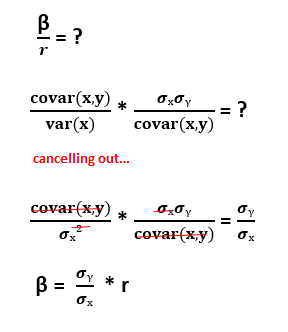Whoa. Beta equals correlation times the ratio of the standard deviations. The significance of that insight is about to become clear as we move from our general use of regression to the familiar CAPM regression. From the CAPM formula we can derive the basis of hedge ratios and more! We have done all the heavy lifting at this point. The reward will be a set of simple, handy formulas that have served me throughout my trading career. Let’s continue. ## From Regression To CAPM The famous CAPM pricing equation is a simple linear regression stipulating that the return of an asset is a function of the risk free rate, a beta to the broader market, plus an error term that represents the security’s own idiosyncratic risk. Rᵢ = Rբ + β(Rₘ – Rբ) + Eᵢ where: Rᵢ = security total return Rբ = risk-free rate β = sensitivity of security’s return to the overall market’s excess return (ie the return above the risk-free rate) Eᵢ = the security’s unique return (aka the error or noise term) Since the risk-free rate is a constant, let’s scrap it to clean the equation up.This is the variance equation for this security:Recall that beta is the vol ratio * correlation:We can use this to factor the “market variance” term.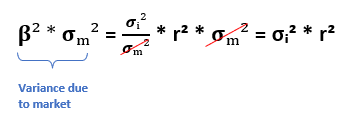Plugging this form of “variance due to the market” back into the variance equation: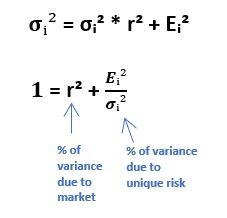This reduces to the prized equation: The “risk remaining” formula which is the proportion of a stock’s volatility due to its own idiosyncratic risk.This makes sense. R2 is the amount of variance in a dependant variable attributable to indepedent variable. If we subtract that proportion from 1 we arrive at the “unexplained” or idiosyncratic variance. By taking the square root of that quantity, we are left with unexplained volatility or “risk remaining”. Let’s use what we’ve learned in a concrete example. ## From CAPM To Hedge Ratios Let’s return to Berkshire vs the SP500. Suppose we are long$10mm worth of BRK.B and want to hedge our exposure by going short SP500 futures.

We want to compute:

1. How many dollars worth of SP500 to get short
2. The “risk remaining” on the hedged portfolio

How many dollars of SP500 do we need to short?

Before we answer this lets consider a few ways we can hedge with SP500.

• Dollar weighting

We could simply sell $10mm worth of SP500 futures which corresponds to our$10mm long in BRK.B. Since Berkshire and the SP500 are a similar volatility this is a reasonable approach. But suppose we were long TSLA instead of BRK.B. Assuming TSLA was sufficiently correlated to the market (say .70 like BRK.B), the SP500 hedge would be “too light”.

Why?

Because TSLA is about 3x more volatile than the SP500. If the SP500 fell 1 standard deviation, we expect TSLA to fall .70 standard deviations. Since TSLA’s standard deviations are much larger than the SP500 we would be tragically underhedged. Our TSLA long would lose much more money than our short SP500 position because we are not short enough dollars of SP500.

• Vol weighting

Dollar weighting is clearly naive if there are large differences in volatility between our long and short. Let’s stick with the TSLA example. If TSLA is 3x as volatile as the SP500 then if we are long $10mm TSLA, we need to short$30mm worth of SP500.

Uh oh.

That’s going to be too much. Remember the correlation. It’s only .70. The pure vol weighted hedge only makes sense if the correlations are 1. If the SP500 drops one standard deviation, we expect TSLA to drop only .70 standard deviations, not a full standard deviation. In this case, we will have made too much money on our hedge, but if the market would have rallied 1 standard deviation our oversized short would have been “heavy”. We would lose more money than we gained on our TSLA long. Again, only partially hedged.

• Beta weighting

Alas, we arrive at the goldilocks solution. We use the beta or slope of the linear regression to weight our hedge. Since beta equals correlation * vol ratio we are incorporating both vol and correlation weighting into our hedge!

I made up numbers vols and correlations to complete the summary tables below. The key is seeing how much the prescribed hedge ratios can vary depending on how you weight the trades.Beta weighting accounts for both relative volatilies and the correlation between names. Beta has a one-to-many relationship to its construction. A beta of .5 can come from:

• A .50 correlation but equal vols
• A .90 correlation but vol ratio of .56
• A .25 correlation but vol ratio of 2

It’s important to decompose betas because the correlation portion is what determines the “risk remaining” on a hedge. Let’s take a look.

How much risk remains on our hedges?

We are long $10,000,000 of TSLA We sell$21,000,000 of SP500 futures as a beta-weighted hedge.

Risk remaining is the volatility of TSLA that is unexplained by the market.

• R2 is the amount of variance in the TSLA position explained by the market.
• 1-R2 is the amount of variance that remains unexplained
• The vol remaining is sqrt(1-R2)

Risk (or vol) remaining = sqrt (1-.72) = 51%

TSLA annual volatility is 45% so the risk remaining is 51% * 45% = 22.95%

22.95% of $10,0000 of TSLA =$2,295,000

So if you ran a hedged position, within 1 standard deviation, you still expect $2,295,000 worth of noise! Remember correlation is symmetrical. The correlation of A to B is the same as the correlation of B to A (you can confirm this by looking at the formula). Beta is not symmetrical because it’s correlation * σdependant / σindependent Yet risk remaining only depends on correlation. So what happens if we flipped the problem and tried to hedge$10,000,000 worth of SP500 with a short TSLA position.

1. First, this is conceptually a more dangerous idea. Even though the correlation is .70, we are less likely to believe that TSLA’s variance explains the SP500’s variance. Math without judgement will impale you on a spear of overconfidence.

2. I’ll work through the example just to be complete.

To compute beta we flip the vol ratio from 3 to 1/3 then multiply by the correlation of .7

Beta of SP500 to TSLA is .333 * .7 = .233

If we are long $10,000,000 of SP500, we sell$2,333,000 of TSLA. The risk remaining is still 51% but it is applied to the SP500 volatility of 15%.

51% x 15% = 7.65% so we expect 7.65% of $10,000,000 or$765,000 of the SP500 position to be unexplained by TSLA.

3. I’m re-emphasizing: math without judgement is a recipe for disaster. The formulas are tools, not substitutes for reasoning.

Changes in Correlation Have Non-Linear Effects On Your Risk

Hedging is tricky. You can see that risk remaining explodes rapidly as correlation falls.

If correlation is as high as .86, you already have 50% risk remaining!In practice, a market maker may:

1. group exposures to the most related index (they might have NDX, SPX, and IWM buckets for example)
2. offset deltas between exposures as they accumulate
3. and hedge the remaining deltas with futures.

You might create risk tolerances that stop you from say being long $50mm worth of SPX and short$50mm of NDX leaving you exposed the underlying factors which differentiate these indices. Even though they might be tightly correlated intraday, the correlation change over time and your risk-remaining can begin to swamp your edge.

The point of hedging is to neutralize the risks you are not paid to take. But hedging is costly. Traders must always balance these trade-offs in the context of their capital, risk tolerances, and changing correlations.

## Review

I walked slowly through topics that are familiar to many investors and traders. I did this because the grout in these ideas often trigger an insight or newfound clarity of something we thought we understood.

This is a recap of important ideas in this post:

• Variance is a measure of dispersion for a single distribution. Covariance is a measure of dispersion for a joint distribution.
• Just as we take the square root of variance to normalize it to something useful (standard deviation, or in a finance context — volatility), we normalize covariance into correlation.
• Intuition for a positive(negative) correlation: if X is N standard deviations above its mean, Y is r * N standard deviations above(below) its mean.
• Beta is r * the vol ratio of Y to X. In a finance context, it allows it allows us to convert a correlation from a standard deviation comparison to a simple elasticity. If beta = 1.5, then if X is up 2%, I expect Y to be up 3%
• Correlation is symmetrical. Beta is not.
• Ris the variance explained by the independent variable. Risk remaining is the volatility that remains unexplained. It is equal to sqrt(1-R2).
• There is a surprising amount of risk remaining even if correlations are strong. At a correlation of .86, there is 50% unexplained variance!
• Don’t compute robotically. Reason > formulas.

Beware.

Least squares linear regression is only one method for fitting a line. It only works for linear relationships. Its application is fraught with pitfalls. It’s important to understand the assumptions in any models you use before they become load-bearing beams in your process.

References:

The table in this post was entirely inspired by Rahul Pathak’s post Anova For Regression.

For the primer on regression and sum of squares I read these 365 DataScience posts in hte following order:

1. The payoff on a single number is 35-1 but there are 38 numbers on an American wheel. Say you place $1 on each number. You will lose$38, but on one number you will be paid $35 plus the$1 you originally bet so you will lose 2/38 of your bankroll. This variance minimizing strategy can be used if you receive a bunch of promotional chips from a casino conditional on you putting them in play
2. I suspect Buffet is far underweighted tech compared to the SP500. I’m no Buffet expert but this exploration is less analyst and more flowing with zoomed out intuition
3. Tyler Vigen is nerd-famous for cataloging them on his amazing site Spurious Correlations
4. I believe this but recognize there’s a lot of noise in the idea of long-run. And while I believe this, it’s not a hill I’d die on because the question of liquidity gets philosophical faster than my brain can keep up, so I realize I can be wrong.